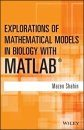United States
£ GBP
EU Shipping Update - read more

## British Wildlife

8 issues per year 84 pages per issue Subscription only

British Wildlife is the leading natural history magazine in the UK, providing essential reading for both enthusiast and professional naturalists and wildlife conservationists. Published eight times a year, British Wildlife bridges the gap between popular writing and scientific literature through a combination of long-form articles, regular columns and reports, book reviews and letters.

Subscriptions from £32 per year

## Conservation Land Management

4 issues per year 44 pages per issue Subscription only

Conservation Land Management (CLM) is a quarterly magazine that is widely regarded as essential reading for all who are involved in land management for nature conservation, across the British Isles. CLM includes long-form articles, events listings, publication reviews, new product information and updates, reports of conferences and letters.

Subscriptions from £22 per year

# Explorations of Mathematical Models in Biology with MATLAB

Textbook
By: Mazen Shahin(Author)
320 pages
Click to have a closer look
•ISBN: 9781118032121 Hardback Mar 2014 Not in stock: Usually dispatched within 6 days
£96.95
#207876

As biology increasingly depends on data, algorithms, and models, it has become necessary to use a computing language, such as the user-friendly MATLAB, to focus more on building and analyzing models as opposed to configuring tedious calculations. Explorations of Mathematical Models in Biology with MATLAB provides an introduction to model creation using MATLAB, followed by the translation, analysis, interpretation, and observation of the models.

With an integrated and interdisciplinary approach that embeds mathematical modeling into biological applications, Explorations of Mathematical Models in Biology with MATLAB illustrates numerous applications of mathematical techniques within biology, ecology, and environmental sciences. Featuring a quantitative, computational, and mathematical approach, the book includes:

- Examples of real-world applications, such as population dynamics, genetics, drug administration, interacting species, and the spread of contagious diseases, to showcase the relevancy and wide applicability of abstract mathematical techniques
- Discussion of various mathematical concepts, such as Markov chains, matrix algebra, eigenvalues, eigenvectors, first-order linear difference equations, and nonlinear first-order difference equations
- Coverage of difference equations to model a wide range of real-life discrete time situations in diverse areas as well as discussions on matrices to model linear problems
- Solutions to selected exercises and additional MATLAB codes

Explorations of Mathematical Models in Biology with MATLAB is an ideal textbook for upper-undergraduate courses in mathematical models in biology, theoretical ecology, bioeconomics, forensic science, applied mathematics, and environmental science. The book is also an excellent reference for biologists, ecologists, mathematicians, biomathematicians, and environmental and resource economists.

## Contents

PREFACE ix

ACKNOWLEDGMENTS xiii

1 OVERVIEW OF DISCRETE DYNAMICAL MODELING AND MATLAB 1

1.1 Introduction to Modeling and Difference Equations / 1

1.2 The Modeling Process / 8

1.3 Getting Started with MATLAB / 13

2 MODELING WITH FIRST-ORDER DIFFERENCE EQUATIONS 28

2.1 Modeling with First-Order Linear Homogenous Difference Equations with Constant Coefficients / 28

2.2 Modeling with Nonhomogenous First-Order Linear Difference Equations / 42

2.3 Modeling with Nonlinear Difference Equations: Logistic Growth Models / 58

2.4 Logistic Equations and Chaos / 74

3 MODELING WITH MATRICES 85

3.1 Systems of Linear Equations Having Unique Solutions / 85

3.2 The Gauss-Jordan Elimination Method with Models / 99

3.3 Introduction to Matrices / 119

3.4 Determinants and Systems of Linear Equations / 147

3.5 Eigenvalues and Eigenvectors / 160

3.6 Eigenvalues and Stability of Linear Models / 185

4 MODELING WITH SYSTEMS OF LINEAR DIFFERENCE EQUATIONS 195

4.1 Modeling with Markov Chains / 195

4.2 Age-Structured Population Models / 219

4.3 Modeling with Second-Order Linear Difference Equations / 231

5 MODELING WITH NONLINEAR SYSTEMS OF DIFFERENCE EQUATIONS 249

5.1 Modeling of Interacting Species / 249

5.2 The SIR Model of Infectious Disease / 264

5.3 Modeling with Second-Order Nonlinear Difference Equations / 270

REFERENCES 277

INDEX 279

## Biography

Mazen Shahin, PhD, is Professor in the Department of Mathematical Sciences at Delaware State University. He has extensive background and experience in designing interdisciplinary instructional materials that integrate mathematics and other disciplines, such as biology, ecology, and finance. Dr. Shahin's research interests include boundary value problems, dynamical systems, impulsive differential equations, and mathematics education.

Textbook
By: Mazen Shahin(Author)
320 pages